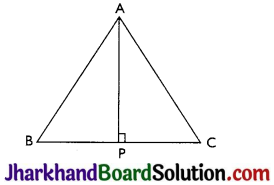# JAC Class 9 Maths Solutions Chapter 7 Triangles Ex 7.3

Jharkhand Board JAC Class 9 Maths Solutions Chapter 7 Triangles Ex 7.3 Textbook Exercise Questions and Answers.

## JAC Board Class 9th Maths Solutions Chapter 7 Triangles Ex 7.3

Page-128

Question 1.
∆ABC and ∆DBC are two isosceles triangles on the same base BC and vertices A and D are on the same side of BC (see Fig). If AD is extended to intersect BC at P, show that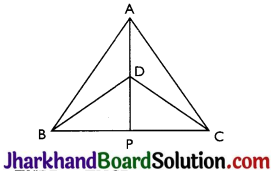(i) ΔABD ≅ ΔACD
(ii) ΔABP ≅ ΔACP
(iii) AP bisects ∠A as well as ∠D.
(iv) AP is the perpendicular bisector of BC.
Given: ΔABC and ΔDBC are two isosceles triangles.
Proof: (i) In ΔABD and ΔACD,
AB = AC (ΔABC is isosceles)
BD = CD (ΔDBC is isosceles)
Therefore, ΔABD ≅ ΔACD by SSS congruence criterion.

(ii) In ΔABP and ΔACP,
AP = AP (Common)
∠PAB = ∠PAC (ΔABD ≅ ΔACD so by CPCT)
AB = AC (ΔABC is isosceles)
Therefore, ΔABP ≅ ΔACP by SAS congruence criterion.

(iii) ∠PAB = ∠PAC by CPCT as ΔABD ≅ ΔACD.
AP bisects ∠A. ………..(i)
Also, in ΔBPD and ΔCPD,
PD = PD (Common)
BD = CD (ΔDBC is isosceles)
BP = CP (ΔABP ≅ ΔACP so by CPCT)
Therefore, ΔBPD ≅ ΔCPD by SSS congruence criterion.
Thus, ∠BDP = ∠CDP by CPCT. …(ii) By (i) and (ii) we can say that AP bisects ∠A as well as ∠D.

(iv) ∠BPD = ∠CPD (by CPCT as ΔBPD = ΔCPD)
and BP = CP …(hi)
Also, ∠BPD + ∠CPD = 180° (BC is a straight line)
⇒ 2∠BPD = 180°
⇒ ∠BPD = 90° …(iv)
From (i) and (ii),
AP is the perpendicular bisector of BC.Question 2.
AD is an altitude of an isosceles triangle ABC in which AB = AC. Show that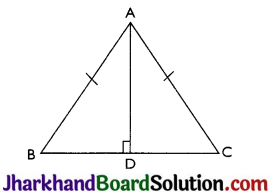Proof: (i) In ΔABD and ΔACD,
AB = AC (Given)
Therefore, ΔABD ≅ ΔACD by RHS congruence criterion.
Now,
BD = CD (by CPCT)

Question 3.
Two sides AB and BC and median AM of one triangle ABC are respectively equal to sides PQ and QR and median PN of ΔPQR (see Fig). Show that: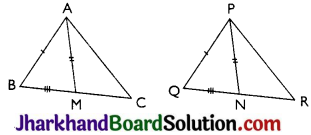(i) ΔABM ≅ ΔPQN
(ii) ΔABC ≅ ΔPQR
Given: AB = PQ, BC = QR and AM = PN
Proof:
(i) $$\frac{1}{2}$$ BC = BM and $$\frac{1}{2}$$ QR = QN (AM and PN are medians)
BC = QR (Given)
⇒ $$\frac{1}{2}$$ BC = $$\frac{1}{2}$$ QR
⇒ BM = QN

In ΔABM and ΔPQN,
AM = PN (Given)
AB = PQ (Given)
BM = QN (Proved above)
Therefore, ΔABM ≅ ΔPQN by SSS congruence criterion.

(ii) In ΔABC and ΔPQR,
AB = PQ (Given)
∠ABC = ∠PQR
(AABM = APQN so by CPCT) BC = QR (Given)
Therefore, ΔABC ≅ ΔPQR by SAS congruence criterion.Question 4.
BE and CF are two equal altitudes of a triangle ABC. Using RHS congruence rule, prove that the triangle ABC is isosceles.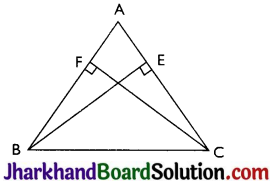Ans. Given: BE and CF are two equal altitudes.
Proof: In ABEC and ACFB,
∠BEC = ∠CFB = 90° (Altitudes)
BC = CB (Common)
BE = CF (Given)
Therefore, ΔBEC ≅ ΔCFB by RHS congruence criterion.
Now,
∠C = ∠B (by CPCT)
Thus, AB = AC (sides opposite to the equal angles are equal.)
Therefore, ΔABC is isosceles.

Question 5.
ABC is an isosceles triangle with AB = AC. Draw AP ⊥ BC to show that ∠B = ∠C.